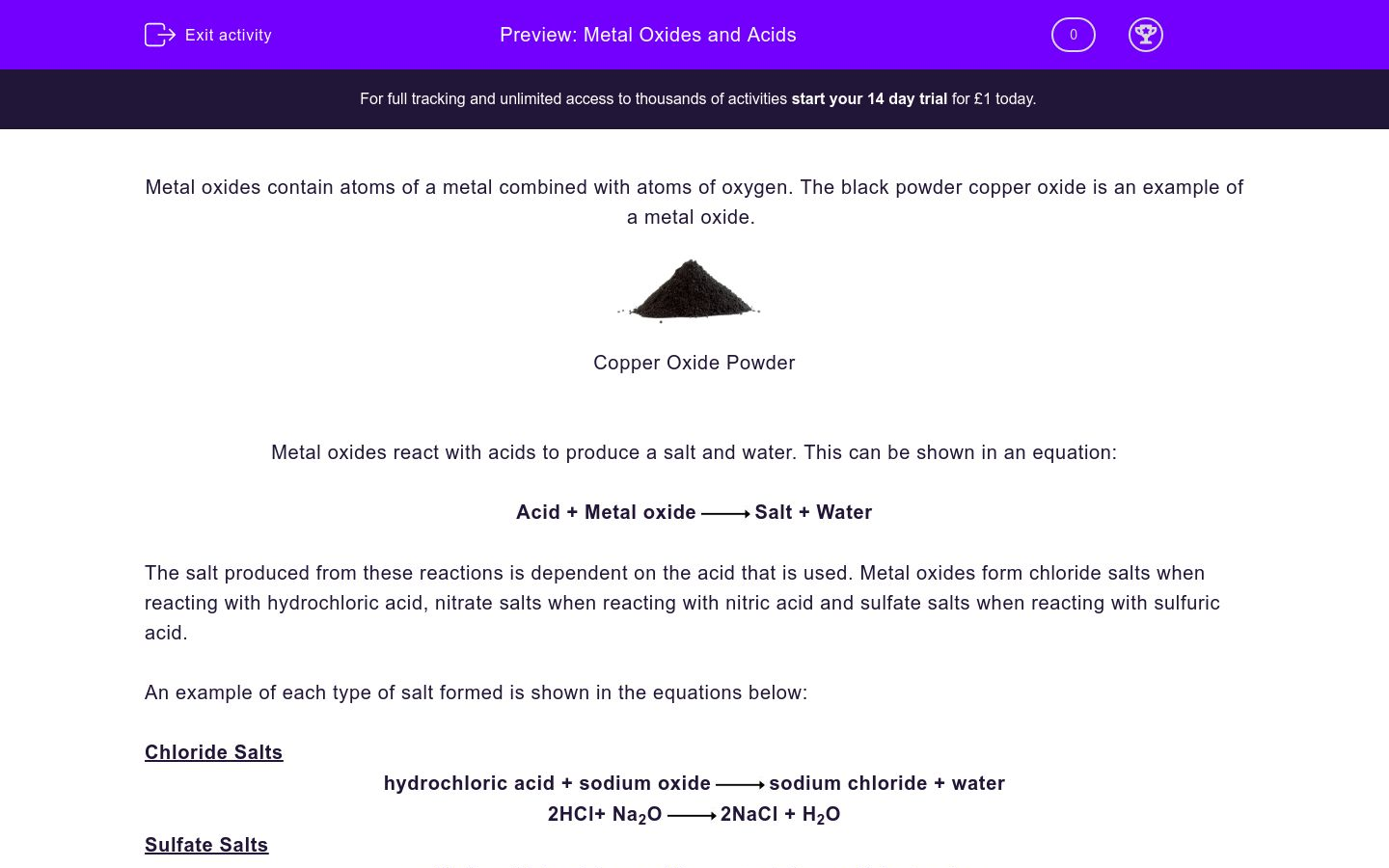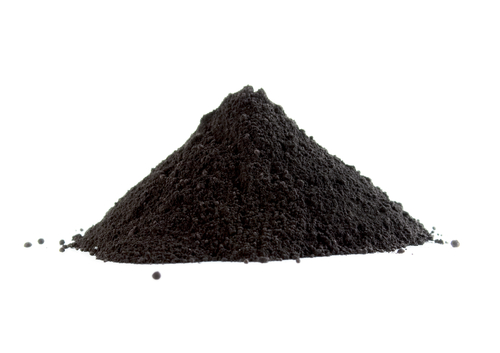# Metal Oxides and Acids

In this worksheet, students will look at how metal oxides react with acids and what is produced by these reactions.Key stage:  KS 3

Curriculum topic:   Chemistry: The Periodic Table

Curriculum subtopic:   pH: Metal and Non-metal Oxides

Difficulty level:### QUESTION 1 of 10

Metal oxides contain atoms of a metal combined with atoms of oxygen. The black powder copper oxide is an example of a metal oxide.Copper Oxide Powder

Metal oxides react with acids to produce a salt and water. This can be shown in an equation:

Acid + Metal oxideSalt + Water

The salt produced from these reactions is dependent on the acid that is used. Metal oxides form chloride salts when reacting with hydrochloric acid, nitrate salts when reacting with nitric acid and sulfate salts when reacting with sulfuric acid.

An example of each type of salt formed is shown in the equations below:

Chloride Salts

hydrochloric acid + sodium oxidesodium chloride + water

2HCl+ Na2O2NaCl + H2O

Sulfate Salts

sulfuric acid + calcium oxidecalcium sulfate + water

H2SO4+ CaOCaSO+ H2O

Nitrate Salts

nitric acid + copper oxidecopper nitrate + water

2HNO3+ CuOCu(NO3)2 + H2O

It is important to learn the formulae of the acids, as they are the three most commonly used acids in the science lab.  Use this information in this activity.

What are the two products of the reaction between a metal oxide and an acid?

a salt and hydrogen

a salt and oxygen

a metal oxide and water

a salt and water

Which of the following is not the correct name of an acid?

hydrochloric acid

sulfuric acid

celtic acid

nitric acid

Match each of the formulae below to the correct acid name using the letters A, B and C:

A = HCl

B = HNO3

C = H2SO4

## Column B

A
nitric acid
B
hydrochloric acid
C
sulfuric acid

Identify the acid that can be used to produce the salts shown.

 Hydrochloric acid Sulfuric acid Nitric acid Sodium chloride magnesium nitrate potassium chloride aluminium sulfate ammonium nitrate zinc chloride

Which of the following salts is not produced from nitric acid?

calcium sulfate

potassium nitrate

sodium nitrate

magnesium nitrate

Complete the equation by typing the name of the missing chemical into the text box below.

Calcium oxide + Hydrochloric acid__________  __________ + Water

Complete the following equation by typing the name of the missing compound into the text box below.

______________  __________ + sodium oxidesodium chloride + Water

Complete the equation by typing the name of the metal oxide used in the reaction into the text box below.

__________  ________ + Nitric acidSodium nitrate + Water

• Question 1

What are the two products of the reaction between a metal oxide and an acid?

a salt and water
EDDIE SAYS
The reaction between an acid and a metal oxide produces a salt and water.
• Question 2

Which of the following is not the correct name of an acid?

celtic acid
EDDIE SAYS
Nitric, sulfuric and hydrochloric acids are all commonly used in the school lab and as such it is important to learn both their names and their formulae.
• Question 3

Match each of the formulae below to the correct acid name using the letters A, B and C:

A = HCl

B = HNO3

C = H2SO4

## Column B

A
hydrochloric acid
B
nitric acid
C
sulfuric acid
EDDIE SAYS
You may well have nailed this already, but look at the negative ion in each case, e.g. NO3 which is a nitrate ion and so is clearly the hydrogen 'salt' of nitric acid. In your head you could even think of it as Hydrogen Nitrate - then the metal salt produced flows effortlessly from it.
• Question 4

Identify the acid that can be used to produce the salts shown.

 Hydrochloric acid Sulfuric acid Nitric acid Sodium chloride magnesium nitrate potassium chloride aluminium sulfate ammonium nitrate zinc chloride
EDDIE SAYS
The name of the salt produced in these reactions gives clues to the acid used. It's easy once you know how: if sulfuric acid, in your head, becomes Hydrogen Sulfate, then clearly aluminium sulfate must come from that. It could hardly come from 'Hydrogen Nitrate', could it?
• Question 5

Which of the following salts is not produced from nitric acid?

calcium sulfate
EDDIE SAYS
This one should be fairly straight forward. The clue is in the name.
• Question 6

Complete the equation by typing the name of the missing chemical into the text box below.

Calcium oxide + Hydrochloric acid__________  __________ + Water

calcium chloride
EDDIE SAYS
The metal from the oxide combines with the the acid to form a salt. So, in this case, the salt must be calcium chloride (CaCl2) as the oxide is CaO and the acid is HCl.
• Question 7

Complete the following equation by typing the name of the missing compound into the text box below.

______________  __________ + sodium oxidesodium chloride + Water

hydrochloric acid
EDDIE SAYS
Word equations are used very often in science - remember the reactants are on the left and the products on the right!
• Question 8

Complete the equation by typing the name of the metal oxide used in the reaction into the text box below.

__________  ________ + Nitric acidSodium nitrate + Water

sodium oxide
EDDIE SAYS
The 'sodium' in the salt name suggests that sodium oxide must have been used in this reaction.
---- OR ----